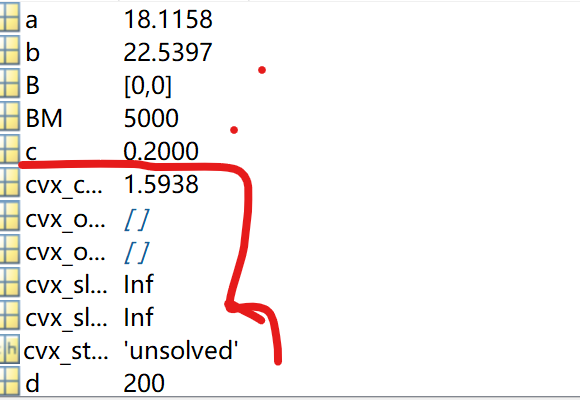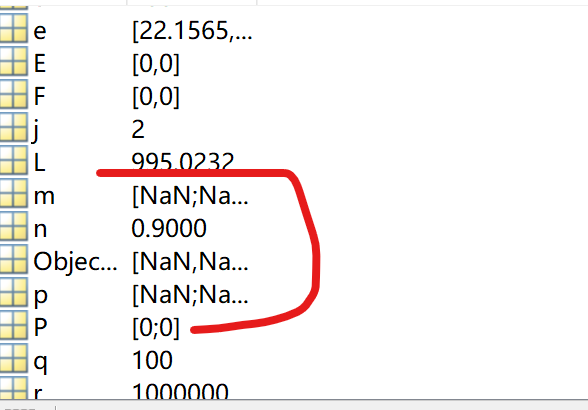# Code error, ask for directions

0<= B(1)-r*(log(3*q+p(1)L)/(log(2)3r)+B(1)/r+log(3q)/(log(2)3r))+log(1+p(1)*L/q)/(log(2)*3)<= BM;

cvx_begin
variable p(2) nonnegative;%非负
variable m(2) nonnegative;%非负
minimize((F(1)+V)(c/r)(3000000-(log(1+(p(1)L)/300)/log(2))+3B(1))+(E(1)+V)p(1)+E(1)e+(V(1-n)-E(1))m(1)+nE(1)m(2)+(F(2)+V)(c/r)(3000000-(log(1+(p(2)L)/300)/log(2))+3B(2))+(E(2)+V)*p(2)+E(2)e+(V(1-n)-E(2))m(2)+nE(2)*m(1))
subject to

``````   P(1) <= E(1)+n*m(2)-m(1);
P(2) <= E(2)+n*m(1)-m(2);
0<= B(1)-r*(log(3*q+p(1)*L)/(log(2)*3*r)+B(1)/r+log(3*q)/(log(2)*3*r))+log(1+p(1)*L/q)/(log(2)*3)<= BM;
0<= B(2)-r*(log(3*q+p(2)*L)/(log(2)*3*r)+B(2)/r+log2(3*q)/(log(2)*3*r))+log2(1+p(2)*L/q)/(log(2)*3)<= BM;
``````

cvx_end

thanks,I am waiting for directions

How have you proven that the RHS of that inequality constraint is concave?

I will assume that constraint is non-convex,until you prove otherwise.

sir,After removing the nonconvex constraint, we get：Your objective function is not a scalar.

Remove `minimize( )` from around the objective function and enter it at the command line. CVX should tell you what type of object it is, including the dimensions. Presumably you will see it is not a scalar. Your objective must evaluate to s scalar. If you change `p` and `m` to be 2 by 1 numerically populated MATLAB variables, such as `ones(2,1)``, your objective function should evaluate in MATLAB to s scalar. if it doesn’t you need to fix something.

thanks very much .
I have a problem,I don’t know how to write the function .
I’ve read the user’s manual over and over again, and this is the only relevant information I can find. I still don’t know how to express this problem in the form that CVX can solve. I am eager for your help.
variable p(6);
variable m(6,6)；
sum((F(j)+V)(c/r)(3000000-(log(1+(p(j)L)/300)/log(2))+3B(j))+(E(j)+V)*p(j)+E(j)e+(V(1-n)-E(j))m1+nE(j)*m2)
j=1:1:6
m(j,j)=0;
m1=sum m(j: ), m2=sum m( :j),

subject to
for j=1:1:6
P(j) <= E(j)+n*sum m( ;j)-sum m(j; );

I can prove that this problem is a convex optimization problem, and the constraints are also convex。Thank you very much for your help

The problem can not be a convex optimization problem unless the objective function evaluates to a real scalar. Can you write out mathematically what the objective function is supposed to be? Is ti a scalar?

I don’t think you want index `j` in the objective function. I don’t even know what the value of `j` is when the minimize statement occurs. perhaps you want a sum(mation) to produce a scalar rather than a vector?

Sorry, I didn’t make it clear. What I want is a scalar and a scalar generated by the form. The objective function is the summation of six scalars obtained by J from 1 to 6.

Where p (6) and m (6,6) are variables.

The other letters represent quantities that are known.The partial quantity is calculated according to the variable value obtained by CVX in the last cycle

Cab you write out a mathematical formula for the objective function, with all varaibles and their dimensions clearly defined

Keep in mind, that even if you can’t figure out the code for a vectorized formula with `sum`, you can always build up the objective in a for loop.

``````cvx_begin
variable x(n)
Objective = 0;
for j=1:n
Objective = Objective + some DCP formula involving x(j)
end
minimize(Objective)
cvx_end``````Your objective function is not a scalar.
Sir, I can’t find my mistake. Can you see where the problem is

For diagnostic purposes, replace

``````variable p(6);
variable m(6,6)
...
minimize(obj)
``````

with

``````p = ones(6,1);
m = ones(6,6);
...
my_objective = obj
whos my_objective
``````

What is the output of
`whos `my_objective` ? This disgnostic program is pure MATLAM; CVX is not involved at all. If`my_objective` is not a scalar, you have not formulated an objective function which evaluate to a scalar. That is a MATLAB or optimization formulation error and has nothing do fo with CVX. If this produces a scalar, then when you go back to the CVX program, that should produce a scalar objective function, hence no error message for not being a scalar.
.

Thank you, sir. After I replace it, I no longer show that error, but there is a new problem. These days is really troubling you, but I really have no other way.
warning: A non-empty cvx problem already exists in this scope.
It is being overwritten.

In cvxprob (line 28)
In cvx_begin (line 41)
In ceshi2 (line 29)
The following error occurred during conversion from CVX to double:
Cannot convert from CVX to double.

To make it clear, I’ve posted the code。

syms V;%非负控制参数
syms t;%时隙
syms p;%发射功率
syms m;%基站之间传递能量
n=0.9;%基站间传输能量的损耗率
G= 18;%
r=1000000;%用户设备传输速率,bit,1Mb/s
c=0.2;%冷却时间的约束，最多占时隙的百分比
d=200;%m,%基站到用户设备的距离,假定同一基站距离用户对的距离是相同的。
q= 100;%噪声功率电平10DB的平方
for V=0:20:100
for t=0:1:5000
L=normrnd(0,5.8);%生成一个正态分布随机数
L=(6.14+20log10(200)+L)18;%路径损耗基站天线增益18DB用户天线增益0DB
if t==0 %冷却时间和能量队列的初始值
F=[0,0,0,0,0,0];
E=[0,0,0,0,0,0];
B=[0,0,0,0,0,0];
end
%重复以下步骤，利用cvx解决优化问题来获得P和m
a= unifrnd(0,20,1,1);%dBm
b=unifrnd(20,40,1,1);%dBm
e =unifrnd (a,b,1,6);%dBm，基站收集的能量均匀分布,生成一个随机数向量
cvx_begin
variable p(6) nonnegative;%非负
variable m(6,6) nonnegative;%非负
Objective = 0;
m1=[0,0,0,0,0,0];
m2=[0,0,0,0,0,0];
for j=1:6
for x=1:6
if x~=j
m1(j)=m1(j)+m(j,x);
end
end
for x=1:6
if x~=j
m2(j)=m2(j)+m(x,j);
end
end
F=F(j);
E=E(j);
B=B(j);
e=e(j);
Objective = Objective + ding(p,m1,m2);%自定义函数ding
end
minimize(Objective)
subject to
for j=1:1:6
for x=1:6
if x~=j
m1(j)=m1(j)+m(j,x);
end
end
for x=1:6
if x~=j
m2(j)=m2(j)+m(x,j);
end
end
P(j) <= E(j)+nm2(j)-m1(j);
end
cvx_end
%更新冷却时间队列长度，更新能量队列长度,更新用户设备数据存储
E1=0;
E2=0;
F1=0;
F2=0;
for j=1:6
for x=1:6
if x~=j
m1=m1+m(j,x);
end
end
for x=1:6
if x~=j
m2(j)=m2(j)+m(x,j);
end
end
F(j)=F(j)+1-log2(3
q+p(j)L)/3r-B(j)/r-log2(3q)/3r-c;
E(j)=E(j)-P(j)+e(j)-m1(j)+nm2(j) ;
B(j)=B(j)-r(log2(3
q+p(j)L)/3r+B(j)/r+log2(3q)/(3r))+log2(1+p(j)L/q)/3;
E1=E1+E(j);%6个基站的电池电量相加
F1=F1+3
F(j);%6个基站分别对应三个视频设备，等待时间相加
end
end
figure(1);
plot(t,E1);
figure(2);
plot(t,F1);
figure(3);
plot(t,M1);
end
function result1 = ding( p,m1,m2 )
result1=(F+V)(c/r)(3r-(log(1+(pL)/300)/log(2))+3B)+(E+V)p+Ee+(V(1-n)-E)m1+nE*m2;
end

You can ignore the warning. It is issued when you do
`cvx_begin`
in a MATLAB session, and before
`cvx_end`
you issue another
`cvx_begin`

The warning is telling you that whatever CVX commands you issued prior to the most recent `cvx_begin` are ignored. I.e., CVX starts over again every time you issue
`cvx_begin`.

If you issue
`cvx_clear`
before another `cvx_begin`, you avoid getting the warning message.

Regarding the error
`Cannot convert from CVX to double. 出错 ceshi2 (line 38) m1(j)=m1(j)+m(j,x);`
that occurs because you are making `m1` into a CVX expression, and you need to declare it as

``````expression m1(6)
expression m2(6)
``````

not

``````m1=[0,0,0,0,0,0]`
m2=[0,0,0,0,0,0];
``````

Sir, after I replace the program,I can run it and get an image with no data but coordinates.

In cvx_end (line 22)
In ceshi2 (line 74)
However, running the original program later will still prompt me that there is no scalar. What could be the reason for this?

Your objective function is not a scalar.

cvx_end

I have no idea what programs you ran.

Sir,sorry, I didn’t make it clear.I’m very sorry to take up your time.
I run the diagnostic program you mentioned the other day, as follows.
For diagnostic purposes, replace

``````variable p(6);
variable m(6,6)
...
minimize(obj)
``````

with

``````p = ones(6,1);
m = ones(6,6);
...
my_objective = obj
whos my_objective
``````

I can run it and get an image with no data but coordinates.

In cvx_end (line 22)
In ceshi2 (line 74)

However, running the original program later will still prompt me that there is no scalar. What could be the reason for this?

Your objective function is not a scalar.

cvx_end
For the convenience of explanation, the original code is attached：

syms V;%非负控制参数
syms t;%时隙
n=0.9;%基站间传输能量的损耗率
G= 18;%
r=1000000;%用户设备传输速率,bit,1Mb/s
c=0.2;%冷却时间的约束，最多占时隙的百分比
d=200;%m,
q= 100;%噪声功率电平10DB的平方
for V=0:20:100
for t=0:1:5000
L=normrnd(0,5.8);%生成一个正态分布随机数
L=(6.14+20log10(200)+L)18;
if t==0 %冷却时间和能量队列的初始值
F=[0,0,0,0,0,0];
E=[0,0,0,0,0,0];
B=[0,0,0,0,0,0];
end
a= unifrnd(0,20,1,1);%dBm
b=unifrnd(20,40,1,1);%dBm
e =unifrnd (a,b,1,6);%dBm，
cvx_clear
cvx_begin
variable p(6) nonnegative;%非负
variable m(6,6) nonnegative;%非负
Obj = 0;
expression m1(6)
expression m2(6)
for j=1:6
for x=1:6
if x~=j
m1(j)=m1(j)+m(j,x);
end
end
for x=1:6
if x~=j
m2(j)=m2(j)+m(x,j);
end
end
F0=F(j);
E0=E(j);
B0=B(j);
e0=e(j);
p0=p(j);
Obj = Obj + (F0+V)
(c/r)
(3r-(log(1+(p0L)/300)/log(2))+3B0)+(E0+V)p0+E0e0+(V(1-n)-E0)m1+nE0m2;
end
minimize(Obj)
subject to
for j=1:1:6
for x=1:6
if x~=j
m1(j)=m1(j)+m(j,x);
end
end
for x=1:6
if x~=j
m2(j)=m2(j)+m(x,j);
end
end
p(j) <=E(j)+n
m2(j)-m1(j);
end
cvx_end
E1=0;
F1=0;
m3=[0,0,0,0,0,0];
m4=[0,0,0,0,0,0];
for j=1:6
for x=1:6
if x~=j
m3(j)=m3(j)+m(j,x);
end
end
for x=1:6
if x~=j
m4(j)=m4(j)+m(x,j);
end
end
F(j)=F(j)+1-log2(3q+p(j)L)/(3r)-B(j)/r+log2(3q)/(3r)-c;
E(j)=E(j)-p(j)+e(j)-m3(j)+n
m4(j) ;
B(j)=B(j)-r*(log2(3q+p(j)L)/(3r)+B(j)/r-log2(3q)/(3*r))+log2(1+p(j)L/q)/3;
E1=E1+E(j);
F1=F1+3
F(j);
end
end
figure(1);
plot(V,E1);
figure(2);
plot(V,F1);

The reason for doing the diagnostic version is to look art the result of
`whos my_objective`
Is it a scalar? If not, you need to fix your program until it becomes a scalar.

Please help me to see, run the diagnostic program to get such a result, indicating whether it is scalar? What should I modify next?

That shows that what would be your objective function in CVX is 6 by 1 vector. it needs to be size 1x1 .(i.e.,1 by 1, which is a scalar).

The objective function of an optimization problem in CVX must evaluate to a real scalar. Do you perhaps want to sum the elements of the 6 by 1 vector, which would result in a scalar? The readers of the forum are not mind-readers (at least I am not), so we don’t know what your model is intended to be.